# Forest ranger

Forest ranger watching from the hide deers. At one time point, he can see six deers. How many maximum deers can be seen by three rangers at the same time?

n =  6

### Step-by-step explanation:

$n=6=6$Did you find an error or inaccuracy? Feel free to write us. Thank you!## Related math problems and questions:

• 3 cats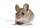3 cats eat 3 mice in 3 days. How many mice will be eaten by 10 cats in 10 days?
• Cents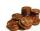Julka has 3 cents more than Hugo. Together they have 27 cents. How many cents has Julka and how many Hugo?
• Number train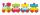The numbers 1,2,3,4,5,6,7,8 and 9 traveled by train. The train had three cars and each was carrying just three numbers. No. 1 rode in the first carriage, and in the last carriage was all odd numbers. The conductor calculated sum of the numbers in the firs
• Expression plus minusEvaluate expression: (-1)2 . 12 – 6 : 3 + (-3) . (-2) + 22 – (-3) . 2
• Dog II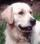The journey from a rangers' home to the town is 6 km. Mr. ranger started from his home and went 3 km per hour. His women came from the town and also went 3 km per hour. With ranger started dog and runs in 12 km in 1 hour. The dog went to the ranger's woma
• Minutes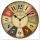Determine the difference in minutes: T1 = 2 3/20 h T2 = 2.3 h
• Expression 1What is 7+8-(5×2)+5-4+(6×(5-3)+6)-(8+10)-7+6?
• Evaluate 11Multiply the quotient of 6 and 2 by 3, then add 1. Add 1 and 6, then divide by 2 and multiply by 3. Divide 6 by the product of 2 and 3, then add 1.
• FractionsSort fractions z1 = (6)/(11); z2 = (10)/(21); z3 = (19)/(22) by its size. Result write as three serial numbers 1,2,3.
• Five mechanicsFive mechanics will make a car for some time. How many cars can 30 mechanics produce at the same time?
• In the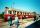In the 6th class, the average half-year mark in physics was 1.7. At the end of the year, Maťo improved his mark from 2 to 1, Ivanka from 3 to 2, and Elenka from 4 to 2. Pavlík worsened his mark from 3 to 4. The average mark of the whole class thus improve
• Czech crownsOldrich has one crown. Peter has five crowns coin, a two crown coin and a one-crown coin. Radek has twenty-crown banknote, ten banknote and five-crown coin. The boys got one fifty-one crown and one crown coin. How can they share the money fairly when they
• PencilsEmil has 23 pencils in a pencil case, 18 of which were broken. Emily 9 trims, 2 breaks, 4 trims and goes to school. When he returned from school, he has broken 5 pencils. How many pencils have trimmed at school?
• Cats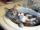Two cats caught two mice in two days. How many mouses will catch 6 cats for 6 days?
• Three cats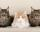If three cats eat three mice in three minutes, after which time 90 cats eat 90 mice?
• Wallpaper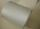Salesman account for 2.3 meters of wallpaper 149.50 Kc. How much cost 1 meter of wallpaper?
• The largest numberFind the largest integer such that: 1. No figures is not repeat, 2. multiplication of every two digits is odd, 3. addition all digits is odd.